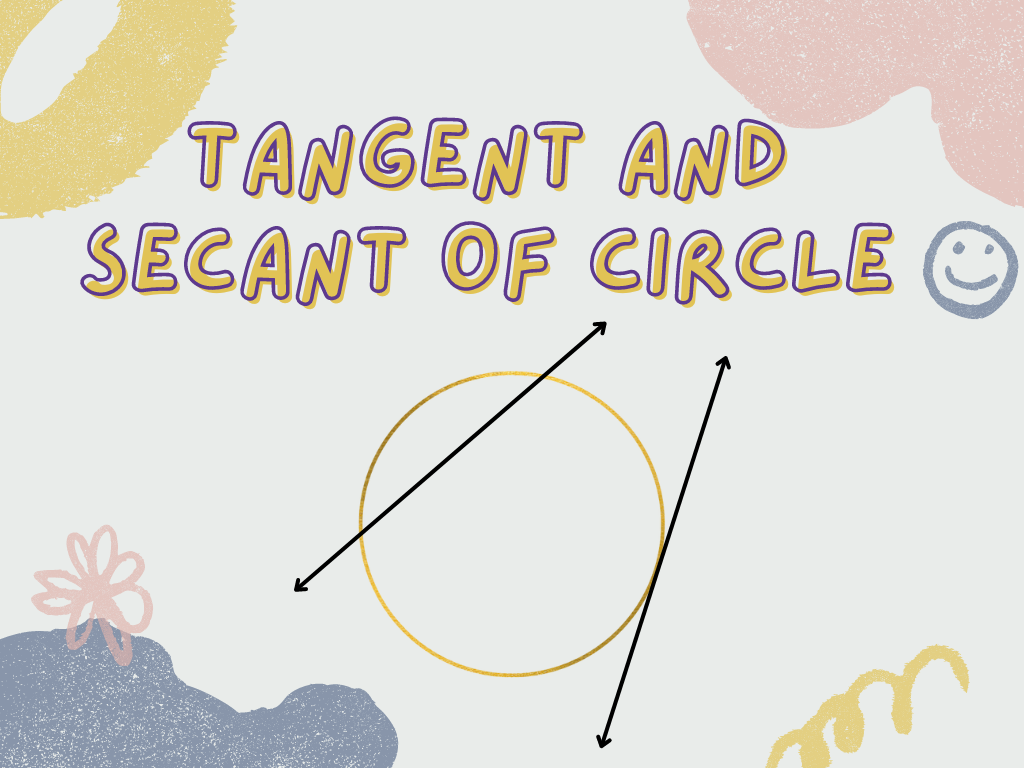## Tangent and Secant of Circle Class 10th

Introduction Definition of Tangent and Secant of Circle Secant line – In a circle, if a line intersects the circle at two points, then that line is called the secant line of the circle. In the above figures, line PQ is a secant line that intersects the circles at points A and B which are …